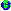StacksForAlice

Note: There also is thex-alice:/lib/data/Stack component in the Alice library, subsuming the module shown here.

(*

Imperative stacks are a mutable data structure.
Every stack contains elements of some type 'a.

- Stack.push adds an element to some stack, while
- Stack.pop remove and returns the lastestly added
element from the stack.
- Stack.new creates and returns a new empty stack.
- Stack.toList returns the list of elements in the
order in which they were added.
- Stack.isEmpty test for emptyness of the stack

The stack implementation shown here is not thread-safe!
Please use the stacks from the library (lib/data/Stack)
for real work.

*)

(*
The signature STACK defines the types of
the functions provided by all stacks.
*)

signature STACK =
sig
type 'a stack
exception Empty
val new     : unit -> 'a stack
val isEmpty : 'a stack -> bool
val pop     : 'a stack -> 'a
val push    : 'a stack -> 'a -> unit
val toList  : 'a stack -> 'a list
end

(*
The structure Stack of signature STACK implements
the functions provided by stacks
*)

structure Stack :> STACK =
struct
type 'a stack = 'a list ref
exception Empty
fun new () = ref []
fun isEmpty s = null (!s)
fun pop s =
case !s of
nil  => raise Empty
| h::t => (s:=t ; h)
fun push s x =
s := x :: !s
fun toList s = !s
end

(*
test stacks

*)

val stack1 = Stack.new ()
val _ = Stack.push (stack1, 1)
val content = Stack.toList stack1
val _ = Stack.pop stack1

val stack2 = Stack.new ()
val _ = Stack.push (stack2, "hello world")

(* raises an exeption

val _ = Stack.pop stack

*)